Prerequisites: install tensorflow 1.0 and scikit-image.

clone this fork of tf-slim somewhere download the pretrained model and put it in tf-models/slim/pretrained/

In :
import tensorflow as tf
slim = tf.contrib.slim
import sys
sys.path.append('/Users/marcotcr/phd/tf-models/slim')
import matplotlib.pyplot as plt
%matplotlib inline
import numpy as np


## Create a predict fn for inception v3, takes in a list of images and returns a matrix of prediction probabilities¶

In :
from nets import inception
from preprocessing import inception_preprocessing

In :
session = tf.Session()

In :
image_size = inception.inception_v3.default_image_size
def transform_img_fn(path_list):
out = []
for f in path_list:
image = inception_preprocessing.preprocess_image(image_raw, image_size, image_size, is_training=False)
out.append(image)
return session.run([out])

In :
from datasets import imagenet

In :
processed_images = tf.placeholder(tf.float32, shape=(None, 299, 299, 3))

In :
import os
with slim.arg_scope(inception.inception_v3_arg_scope()):
logits, _ = inception.inception_v3(processed_images, num_classes=1001, is_training=False)
probabilities = tf.nn.softmax(logits)

checkpoints_dir = '/Users/marcotcr/phd/tf-models/slim/pretrained'
init_fn = slim.assign_from_checkpoint_fn(
os.path.join(checkpoints_dir, 'inception_v3.ckpt'),
slim.get_model_variables('InceptionV3'))
init_fn(session)

In :
def predict_fn(images):
return session.run(probabilities, feed_dict={processed_images: images})


## Let's see the top 5 prediction for some image¶

In :
images = transform_img_fn(['dogs.jpg'])
# I'm dividing by 2 and adding 0.5 because of how this Inception represents images
plt.imshow(images / 2 + 0.5)
preds = predict_fn(images)
for x in preds.argsort()[-5:]:
print x, names[x], preds[0,x]

286 Egyptian cat 0.000892741
242 EntleBucher 0.0163564
239 Greater Swiss Mountain dog 0.0171362
241 Appenzeller 0.0393639
240 Bernese mountain dog 0.829222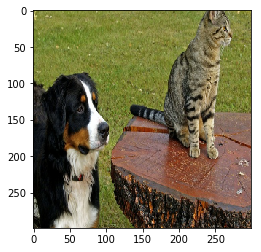In :
image = images

In :
## Now let's get an explanation

In :
from lime import lime_image
import time

In :
explainer = lime_image.LimeImageExplainer()


hide_color is the color for a superpixel turned OFF. Alternatively, if it is NONE, the superpixel will be replaced by the average of its pixels. Here, we set it to 0 (in the representation used by inception model, 0 means gray)

In :
tmp = time.time()
# Hide color is the color for a superpixel turned OFF. Alternatively, if it is NONE, the superpixel will be replaced by the average of its pixels
explanation = explainer.explain_instance(image, predict_fn, top_labels=5, hide_color=0, num_samples=1000)
print time.time() - tmp

440.970395088


Image classifiers are a bit slow. Notice that an explanation in my macbookpro took 7.65 minutes

### Now let's see the explanation for the top class (Bernese mountain dog)¶

We can see the top 5 superpixels that are most positive towards the class with the rest of the image hidden

In :
from skimage.segmentation import mark_boundaries

In :
temp, mask = explanation.get_image_and_mask(240, positive_only=True, num_features=5, hide_rest=True)
plt.imshow(mark_boundaries(temp / 2 + 0.5, mask))

Out:
<matplotlib.image.AxesImage at 0x116ea4cd0>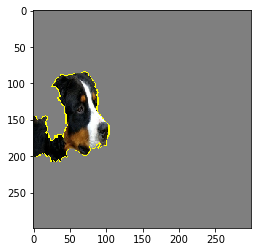Or with the rest of the image present:

In :
temp, mask = explanation.get_image_and_mask(240, positive_only=True, num_features=5, hide_rest=False)
plt.imshow(mark_boundaries(temp / 2 + 0.5, mask))

Out:
<matplotlib.image.AxesImage at 0x1209e18d0>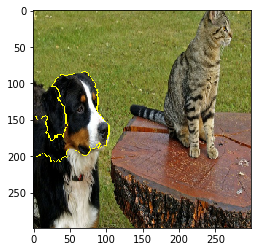We can also see the 'pros and cons' (pros in green, cons in red)

In :
temp, mask = explanation.get_image_and_mask(240, positive_only=False, num_features=10, hide_rest=False)
plt.imshow(mark_boundaries(temp / 2 + 0.5, mask))

Out:
<matplotlib.image.AxesImage at 0x1209e1790>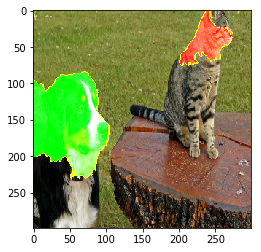Or the pros and cons that have weight at least 0.1

In :
temp, mask = explanation.get_image_and_mask(240, positive_only=False, num_features=1000, hide_rest=False, min_weight=0.1)
plt.imshow(mark_boundaries(temp / 2 + 0.5, mask))

Out:
<matplotlib.image.AxesImage at 0x1209e1850>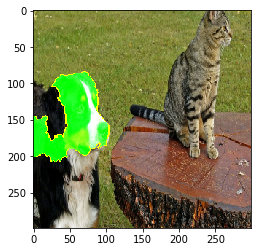### Let's see the explanation for Egyptian cat¶

Most positive towards egyptian cat:

In :
temp, mask = explanation.get_image_and_mask(286, positive_only=True, num_features=5, hide_rest=True)
plt.imshow(mark_boundaries(temp / 2 + 0.5, mask))

Out:
<matplotlib.image.AxesImage at 0x1209e1450>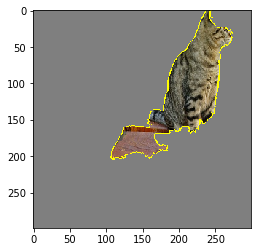Pros and cons:

In :
temp, mask = explanation.get_image_and_mask(286, positive_only=False, num_features=10, hide_rest=False)
plt.imshow(mark_boundaries(temp / 2 + 0.5, mask))

Out:
<matplotlib.image.AxesImage at 0x1209e17d0>